﻿ 重载列车运行工况仿真与试验比较研究 Comparative Study on Simulation and Experiment of Heavy Haul Train Operating Conditions

Open Journal of Transportation Technologies
Vol. 08  No. 02 ( 2019 ), Article ID: 29409 , 9 pages
10.12677/OJTT.2019.82016

Comparative Study on Simulation and Experiment of Heavy Haul Train Operating Conditions

Xingguang Yang, Wei Wei

School of Locomotive and Vehicle Engineering, Dalian Jiaotong University, Dalian Liaoning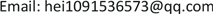Received: Mar. 4th, 2019; accepted: Mar. 18th, 2019; published: Mar. 25th, 2019ABSTRACT

The actual operation of heavy haul trains is affected by many uncertain factors, and the corresponding simulation accuracy is difficult, which makes the longitudinal dynamics research and maneuver optimization difficult. Therefore, the comparative study of simulation accuracy has important practical significance. According to the test data of 20,000 tons of turmeric, the train air brake and longitudinal dynamics simulation system (TABLDSS) is used to calculate the speed and longitudinal dynamics of the train under actual operating conditions, and the simulation results are compared with the test results: the results show that the simulation speed curve agrees well with the test; the speed change trend is basically the same; the speed error is maximum 0.8 km/h; the train running resistance, the locomotive traction/dynamic braking force model have higher accuracy, and the air brake decompression characteristics are basically the same. When the brake is relieved, the tail pressure is basically the same; the brake opening time error is small; the maximum is 0.8 s; the air brake model is accurate; the simulated maximum coupler force occurs in the same position as the test, and the maximum coupler force appears in the train mitigation process. Near the middle locomotive, the braking distance and the hook force error were 2.4% and 4.4%, respectively, and the simulation system was highly accurate. This work provides an advantageous tool for train manipulation optimization.

Keywords:Heavy Haul Train, Braking System, Air Brake, Train Operation, Simulation AnalysisCopyright © 2019 by author(s) and Hans Publishers Inc.1. 前言

Uyulan  采用Matlab-Simulink软件对机车机电系统进行建模仿真，并探讨了机车牵引和制动工况下系统参数对车辆纵向动力学的影响；Mohammadi等  通过建立仿真模型，模拟了重载列车紧急制动情况下，机车位置对列车纵向力的影响；刘华伟等  采用列车纵向动力学仿真程序计算分析了重载列车牵引启动时，不同车钩间隙对列车纵向冲动的影响并提出了列车在启动困难区段的较优启动方式；耿志修  通过建立重载列车运行仿真计算模型，研究了大秦线2万t不同编组组合列车的牵引、制动等技术参数，为大秦线组织重载列车试验、制订合理操纵方法等提供了技术依据；董克毓  分析了万吨列车在神朔段的实际操作情况，利用纵向动力学仿真系统对司机操纵进行优化，通过改变制动初速度、机车电制动力等参数，减少了列车循环制动次数；魏伟  开发了基于气体流动理论的列车空气制动与纵向动力学联合仿真程序(TABLDSS)，可以模拟列车实际操纵指令，模拟不同编组列车在给定线路上的运行过程，并仿真分析了两万吨组合列车制动特性，对两万吨列车纵向动力学性能进行了预测  ；该仿真程序在国际列车纵向动力学仿真系统测评中取得优异成绩，测评结果表明TABLDSS仿真程序具有运算速度快、计算精度高等优点  。这些研究都是针对重载列车模型建立和仿真计算分析展开的，但重载列车运行仿真系统是一整套复杂的关联体系，包括机车牵引和动力制动能力、空气制动特性和缓解再充风能力等，而且列车实际运行情况受线路条件、编组方式、制动缓解能力、自然天气等诸多因素影响，相应仿真精度也存在巨大问题。因此，面对诸多影响因素，提高仿真系统准确性，使仿真结果与试验结果更接近才是关键所在。通过仿真系统真实模拟司机操纵指令，仿真列车实际运行概况，在此基础上分析列车纵向动力学性能和列车受力情况，为重载列车安全开行和对司机操纵优化，提供有力依据。

2. 仿真模型建立

2.1. 列车纵向动力学仿真模型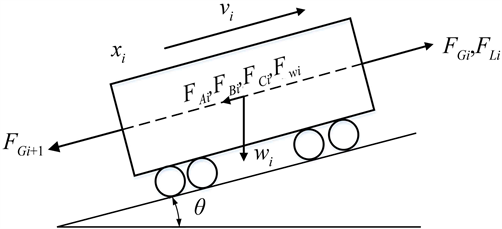Figure 1. The force diagram of a single vehicle

${m}_{i}{\stackrel{¨}{x}}_{i}={F}_{Gi}-{F}_{Gi+1}-{F}_{Ai}-{F}_{Bi}+{F}_{Li}-{F}_{Ci}-{F}_{Wi}\left(i=1,2\cdots ,n\right)$ (1)

${F}_{Gi}=f\left({v}_{ti}-{v}_{ti-1},{s}_{ti}-{s}_{ti-1}\right)\left(i=1,2\cdots ,n\right)$ (2)

${v}_{ti}-{v}_{oi}=\Delta {v}_{i}={\stackrel{¨}{x}}_{i}\Delta t\left(i=1,2\cdots n\right)$ (3)

${s}_{ti}-{s}_{0i}=\Delta s={v}_{i}\Delta t\left(i=1,2\cdots n\right)$ (4)

${v}_{i}=\left({v}_{ti}+{v}_{0i}\right)/2$ (5)

${{w}^{″}}_{0}\text{=}0.9186+0.001v$ (6)

2.2. 空气制动系统模型

$\left\{\begin{array}{l}\frac{\partial \rho }{\partial t}+\rho \frac{\partial u}{\partial x}+u\frac{\partial \rho }{\partial x}+\frac{\rho u}{F}\frac{\text{d}F}{\text{d}x}=0\hfill \\ \frac{\partial u}{\partial t}+u\frac{\partial u}{\partial x}+\frac{1}{\rho }\frac{\partial p}{\partial x}+\frac{4f}{D}\frac{{u}^{2}}{2}\frac{u}{|u|}=0\hfill \\ \frac{\partial p}{\partial t}+u\frac{\partial p}{\partial x}-{a}^{2}\frac{\partial \rho }{\partial t}-{a}^{2}u\frac{\partial \rho }{\partial x}-\left(k-1\right)\rho \left(q+u\frac{4f}{D}\frac{{u}^{2}}{2}\frac{u}{|u|}\right)=0\hfill \end{array}$ (7)

2.3. 机车牵引与动力制动模型

${F}_{\mathrm{max}}\left(\text{kN}\right)=\left\{\begin{array}{cc}\begin{array}{l}760\\ 779.05-3.81v\\ 34541/v\\ 0\end{array}& \begin{array}{l}v<5\\ 5120\end{array}\end{array}$ (8)

HXD1型电力机车牵引特性曲线如图2所示。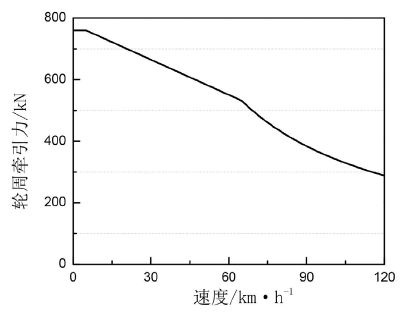Figure 2. HXD1 locomotive traction characteristic curve

HXD1型电力机车最大瞬时动力制动特性公式为：

${F}_{V}=\left\{\begin{array}{cc}\begin{array}{l}153.3v\\ 460\\ 34500/v\\ 0\end{array}& \begin{array}{l}v\le 3\\ 3120\end{array}\end{array}$ (9)

${F}_{V}$$v$ 分别是机车瞬时动力制动力、列车速度。

HXD1型机车瞬时最大动力制动力如图3所示。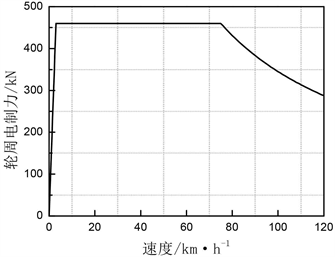Figure 3. HXD1 locomotive braking characteristic curve

3. 仿真与试验结果比较分析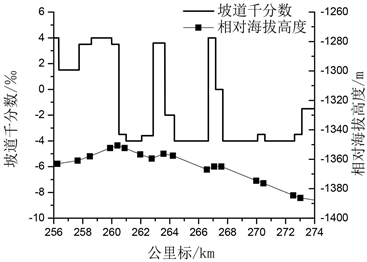Figure 4. The Sanji of the Shuohuang line cover a slope range of 256 km - 274 km/altitude to Lingshou

3.1. 速度比较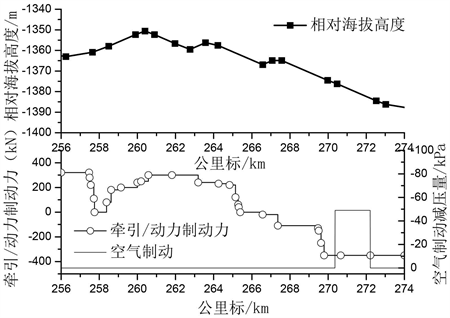Figure 5. Locomotive operating instructions and ramp changes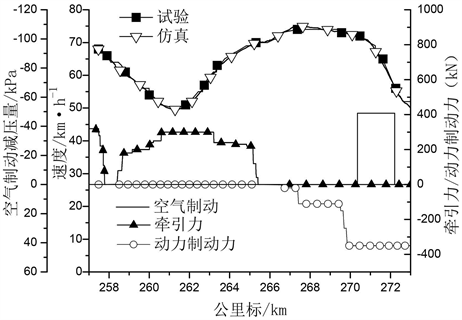Figure 6. The simulation curve of train speed change is compared with the test curveTable 1. The simulation and test results of the key parameters of the train braking mitigation command point are compared

3.2. 空气制动特性比较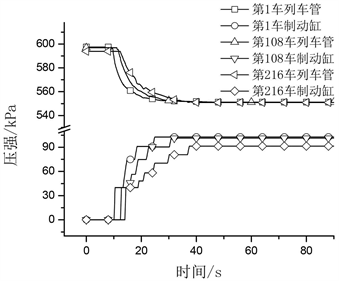Figure 7. Simulation of the pressure curves of train tubes and brake cylinders of cars 1, 108 and 216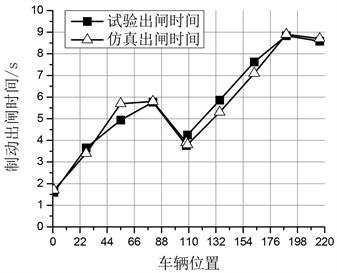Figure 8. The simulation and test of air brake exit time of each parking space are comparedTable 2. Simulation and test results of air brake exit time of each parking space are compared

3.3. 车钩力比较Table 3. Comparison of simulation and test results of common braking and stopping conditions

4. 结论

1) 仿真模拟出的列车运行速度、制动缓解指令点、制动缓解时尾车副风缸压强等关键参数与试验结果吻合很好；仿真速度曲线变化趋势与试验结果基本一致，速度误差最大为0.8 km/h，尾车副风缸压强误差为1 kPa，说明仿真系统中列车运行阻力模型、牵引/动力制动模型和空气制动模型是准确的。

2) 仿真系统可以很好模拟列车开始空气制动列车管、制动缸压强变化过程，仿真空气制动出闸时间分布规律与试验规律吻合程度较高，说明仿真程序空气制动减压特性与试验基本一致，空气制动模型合适。

3) 常用制动停车工况下，仿真制动距离、制动时间和最大车钩力等制动参数与试验结果误差很小，制动距离误差为2.4%；最大车钩力发生位置与试验一致，车钩力误差为4.4%，说明仿真系统精度较高。

Comparative Study on Simulation and Experiment of Heavy Haul Train Operating Conditions[J]. 交通技术, 2019, 08(02): 129-137. https://doi.org/10.12677/OJTT.2019.82016

1. 1. Uyulan, C., Gokasan, M. and Bogosyan, S. (2017) Modeling, Simulation and Slip Control of a Railway Vehicle Integrated with Traction Power Supply. Cogent Engineering, 4, Article ID: 1312680. https://doi.org/10.1080/23311916.2017.1312680

2. 2. Mohammadi, S. and Nasr, A. (2010) Effects of the Power Unit Location on In-Train Longitudinal Forces during Brake Application. International Journal of Vehicle Systems Modelling and Testing, 5, 176. https://doi.org/10.1504/IJVSMT.2010.037125

3. 3. 刘华伟, 王自力. 车钩间隙对重载列车牵引启动纵向冲动的影响[J]. 铁道车辆, 2018, 56(6): 7-9.

4. 4. 耿志修, 李雪峰, 张波. 大秦线重载列车运行仿真计算研究[J]. 中国铁道科学, 2008, 29(2): 89-92.

5. 5. 董克毓，魏伟. 万吨重载列车在神朔段长大下坡道循环制动优化[J]. 大连交通大学学报, 2017, 38(1): 17-20.

6. 6. 魏伟, 赵旭宝, 姜岩. 列车空气制动与纵向动力学集成仿真[J]. 铁道学报, 2012, 34(4): 40-46.

7. 7. 魏伟. 两万吨组合列车制动特性[J]. 交通运输工程学报, 2007, 7(6): 12-16.

8. 8. Wu, Q., Spiryagin, M., Cole, C., et al. (2018) International Benchmarking of Longitudinal Train Dynamics Simulators: Results. Vehicle System Dynamics, 56, 343-365. https://doi.org/10.1080/00423114.2017.1377840

9. 9. 胡杨. 神华铁路万吨重载列车纵向动力学试验与仿真研究[D]: [博士学位论文]. 大连: 大连交通大学, 2017: 73-84.

10. 10. Wei, W., Hu, Y., Wu, Q., et al. (2017) An Air Brake Model for Longitudinal Train Dynamics Studies. Vehicle System Dynamics, 55, 517-533. https://doi.org/10.1080/00423114.2016.1254261

11. 11. 魏伟, 张善荣, 刘庆忠. 长大列车制动系统减压特性的计算机模拟[J]. 大连交通大学学报, 1992, 13(4): 43-49.